monocone

Create monocone antenna on circular ground plane

Description

The monocone object creates a monocone antenna on a circular ground plane. A classical monocone antenna consists of a cone and a ground plane. To increase the bandwidth of the antenna, you can modify the antenna by merging the cone with a circular cylinder. By default, the monocone object creates the modified version.Create a classical monocone antenna (without the cylinder on top) using one of these methods:

• Set the height of the antenna to equal the sum of the cone height and the feed height.

• Set the cone height to equal half of the difference between the total height and the feed height. Then set the radius at the aperture to twice the radius at the junction.

Creation

Description

example

ant = monocone creates a monocone antenna with the feedpoint at the center of the ground plane. The default dimensions are for a resonant frequency of 3.8 GHz.

example

ant = monocone(Name,Value) sets properties using one or more name-value pairs. For example, ant = monocone('Height',0.0560) creates a monocone antenna with a total height of 0.0560 meters.

Properties

expand all

Antenna radii, specified as a three-element real vector with each element unit in meters.

• The first element represents the narrow radius of the cone.

• The second element represents the radius at the junction of the cone and the cylinder.

• The third element represents the radius at the top of the cylinder.

Example: ant.Radii = [6.3300e-04 0.0546 0.0220]

Data Types: double

Total height of the antenna from the ground plane to the aperture of the antenna, specified as a positive scalar in meters.

Example: 'Height',0.0560

Example: ant.Height = 0.0560

Data Types: double

Vertical height of the cone from the apex of the cone to the junction of the cone and the cylinder, specified as a positive scalar in meters.

Example: 'ConeHeight',0.02250

Example: ant.ConeHeight = 0.02250

Data Types: double

Gap between the cone and the ground plane, specified as a positive scalar in meters.

Example: 'FeedHeight',0.0034

Example: ant.FeedHeight = 0.0034

Data Types: double

Width of the feed, specified as a positive scalar in meters.

Example: 'FeedWidth',0.0050

Example: ant.FeedWidth = 0.0050

Data Types: double

Radius of the ground plane, specified as a positive scalar in meters.

Data Types: double

Type of the metal used as a conductor, specified as a metal material object. You can choose any metal from the MetalCatalog or specify a metal of your choice. For more information, see metal. For more information on metal conductor meshing, see Meshing.

Example: m = metal('Copper'); 'Conductor',m

Example: m = metal('Copper'); ant.Conductor = m

Lumped elements added to the antenna feed, specified as a lumpedElement object. You can add a load anywhere on the surface of the antenna. By default, the load is at the feed. For more information, see lumpedElement.

Tilt angle of the antenna, specified as a scalar or vector with each element unit in degrees. For more information, see Rotate Antennas and Arrays.

Example: 'Tilt',90

Example: ant.Tilt = 90

Example: 'Tilt',[90 90],'TiltAxis',[0 1 0;0 1 1] tilts the antenna at 90 degrees about the two axes defined by the vectors.

Note

The wireStack antenna object only accepts the dot method to change its properties.

Data Types: double

Tilt axis of the antenna, specified as:

• Three-element vector of Cartesian coordinates in meters. In this case, each coordinate in the vector starts at the origin and lies along the specified points on the X-, Y-, and Z-axes.

• Two points in space, each specified as three-element vectors of Cartesian coordinates. In this case, the antenna rotates around the line joining the two points in space.

• A string input describing simple rotations around one of the principal axes, 'X', 'Y', or 'Z'.

Example: 'TiltAxis',[0 1 0]

Example: 'TiltAxis',[0 0 0;0 1 0]

Example: ant.TiltAxis = 'Z'

Note

The wireStack antenna object only accepts the dot method to change its properties.

Data Types: double

Object Functions

 coneangle2size Calculates equivalent cone height, broad radius, and narrow radius for cone show Display antenna or array structure; display shape as filled patch axialRatio Axial ratio of antenna beamwidth Beamwidth of antenna charge Charge distribution on metal or dielectric antenna or array surface current Current distribution on metal or dielectric antenna or array surface design Design prototype antenna or arrays for resonance around specified frequency efficiency Radiation efficiency of antenna EHfields Electric and magnetic fields of antennas; Embedded electric and magnetic fields of antenna element in arrays impedance Input impedance of antenna; scan impedance of array mesh Mesh properties of metal or dielectric antenna or array structure meshconfig Change mesh mode of antenna structure optimize Optimize antenna or array using SADEA optimizer pattern Radiation pattern and phase of antenna or array; Embedded pattern of antenna element in array patternAzimuth Azimuth pattern of antenna or array patternElevation Elevation pattern of antenna or array rcs Calculate and plot radar cross section (RCS) of platform, antenna, or array returnLoss Return loss of antenna; scan return loss of array sparameters Calculate S-parameter for antenna and antenna array objects vswr Voltage standing wave ratio of antenna

Examples

collapse all

Create and view a default monocone antenna.

ant = monocone
ant =
monocone with properties:

ConeHeight: 0.0115
Height: 0.0250
FeedHeight: 5.0000e-04
FeedWidth: 5.0000e-04
Conductor: [1x1 metal]
Tilt: 0
TiltAxis: [1 0 0]

show(ant)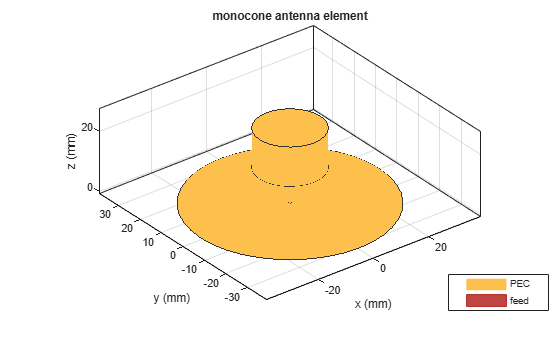Create a monocone antenna with an infinite ground plane.

ant = monocone;
show(ant)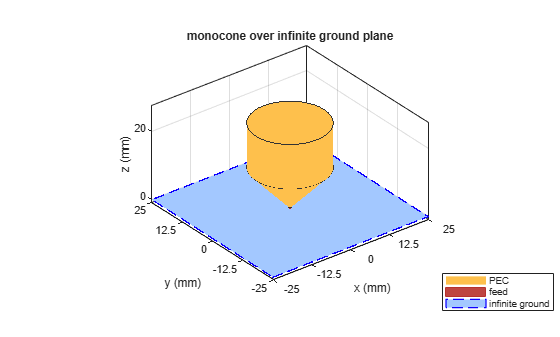Plot the radiation pattern of the monocone antenna for the given frequency.

pattern(ant,3.94e9)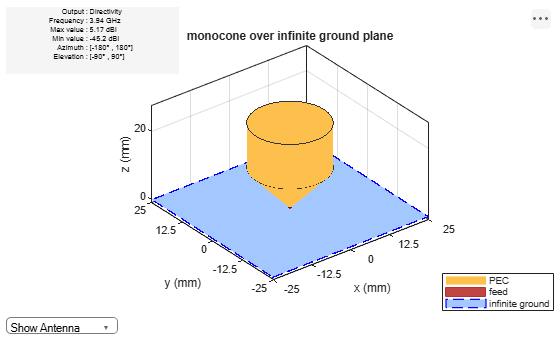Create a classical monocone antenna by setting the total height of the antenna to equal the sum of cone height and feed height.

ant = monocone;
ant.Height = ant.ConeHeight+ant.FeedHeight;
show(ant)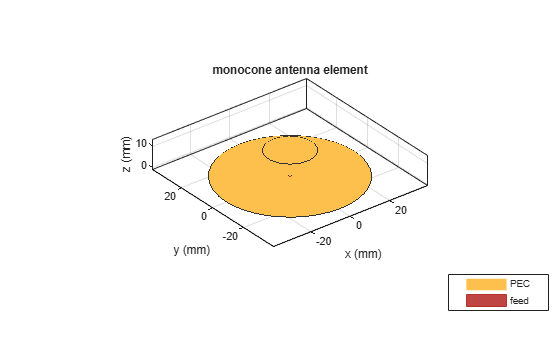Calculate antenna impedance over the given frequency span.

impedance(ant,(1e9:0.1e9:6e9))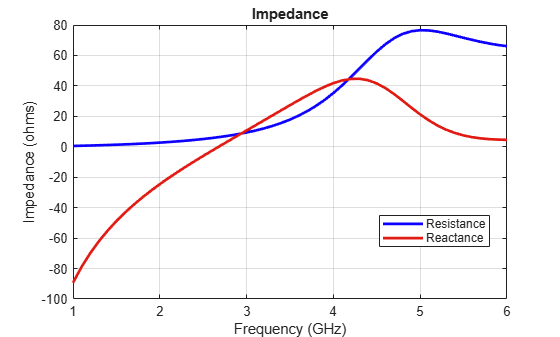McDonald, James L., and Dejan S. Filipovic. “On the Bandwidth of Monocone Antennas.” IEEE Transactions on Antennas and Propagation 56, no. 4 (April 2008): 1196–1201. https://doi.org/10.1109/TAP.2008.919226.